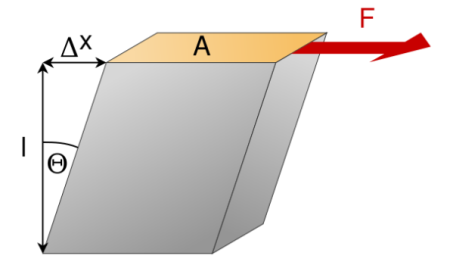# What is shear stress and shear strain?

## Question:

What is shear stress and shear strain?

## Stress and Strain:

Stress and strain are two related concepts in the sciences and engineering that have to do with the deformation of a material when a force is applied to it. There are multiple types of stress and strain that can be applied to a material depending upon how a force is acting on it. Three of the most common are compressive, tensile, and shear stress and strain.

Shear stress and shear strain occur when a force is acting parallel to the cross sectional area of a material. This force is known as a shearing force. Shear stress is the ratio of the shearing force (F) to the cross sectional area (A) to which it is being applied.

{eq}\displaystyle shear\;stress = \frac{F}{A} {/eq}

Shear strain is then the deformation of the material caused by the shear stress it is experiencing. To understand the formula for shear stress we'll be using the following diagram.In this diagram we can tell that a square block is experiencing shear stress as its shape has been altered due to a force acting parallel to its top surface. To express this change in position mathematically we will use the triangle to the left of the block that was created by its deformation. The shear strain of the block is equal to the tangent of Θ, which in turn is equal to the change in position of the top of the block (Δx) divided by the height of the block (l).

{eq}\displaystyle shear\;strain=tan(\Theta) = \frac{\Delta x}{l} {/eq}